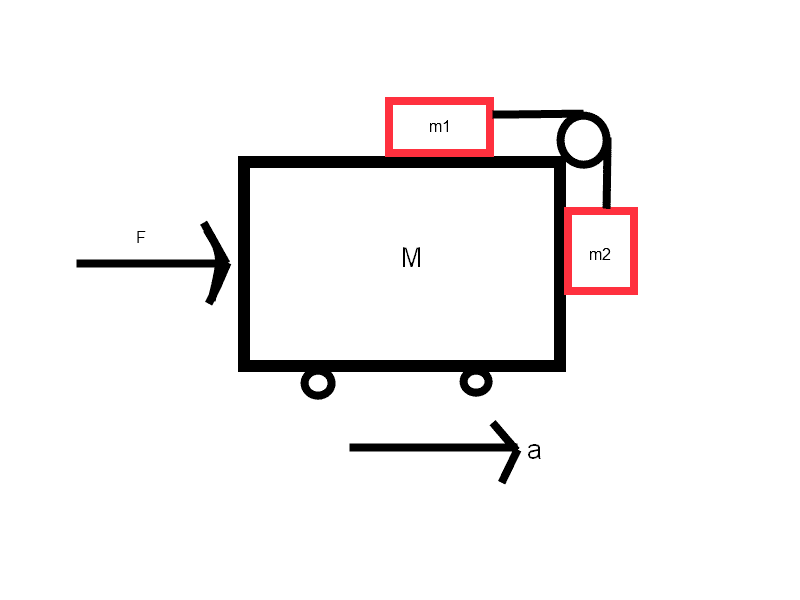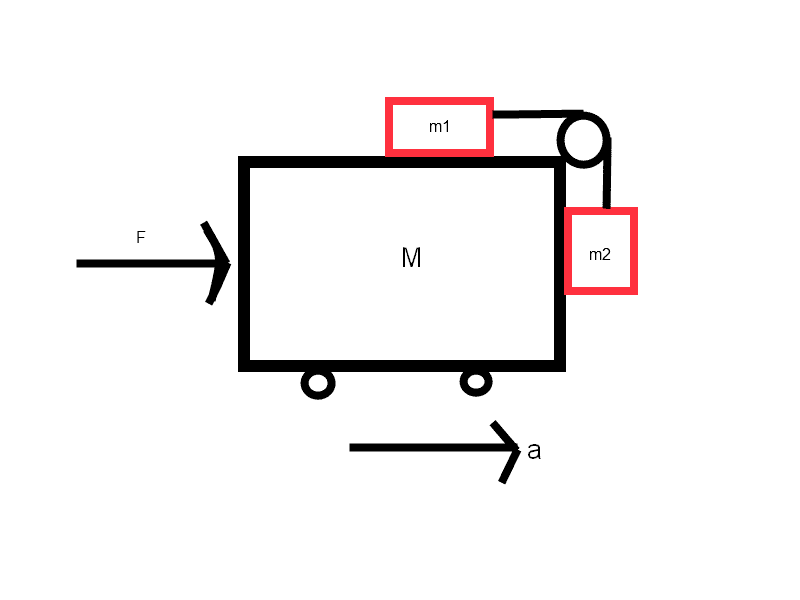# Finding the acceleration of an object

## Homework Statement

In the picture:
http://postimg.org/image/6gdkm5vpx/
There is a force (F) pushing the car to the right
The car's mass is M
There are two other objects with masses m1 and m2 that are connected by a cord with no mass.
There is no friction
In terms of m1, m2 and M find the acceleration needed to keep m1 and m2 from falling down.

## Homework Equations

This is the second Newton's law.
The only equation I use is

Fnet=m*a

## The Attempt at a Solution

I have tried doing this by placing the 3 m's (m1, m2 and M) in their respective Cartesian planes to get the forces of each one, but the part I don't quite understand is which forces I have to put in the formula...
I understand that I need to add the masses:
Fnet=(M+m1+m2)*a
and I understand that Fnet consists of the F force that is affecting the car, but what other?
Do I need to use the tension of the cord that holds m1 and m2 together? or all I do is:
F=(M+m1+m2)*a
So the acceleration would be
F/(M+m1+m2)=a ?

Doc Al
Mentor
I suggest that you begin by analyzing the forces on m2.

tiny-tim
Homework Helper
Welcome to PF!or all I do is:
F=(M+m1+m2)*a
So the acceleration would be
F/(M+m1+m2)=a ?

yesas you say, the only equation you need is F = ma, and the trick is simply which bodies to apply it to
Do I need to use the tension of the cord that holds m1 and m2 together?

yes, and use to it apply F = ma (different F of course!) to the top block and the bottom block (separately) …

what do you get?(btw, there is another way of doing it, by using the fictitious force in the non-inertial rest frame of the car …

if the car is stationary, in which direction would the "effective gravity" have to be for the two blocks not to slide?)

yes, and use to it apply F = ma (different F of course!) to the top block and the bottom block (separately) …

Well the forces in each mass are:
m2: T(tension) and W (weight)
The tension in this case is equal to the weight which is mass*gravity
So T=m*g
and in m1 the forces are weight, Normal force (I don't know how it's called in English but I hope you understand) and the tension which is m2*g, there is no acceleration in Y so I don't need the weight or normal force.

Do I need to use this information to find the car's acceleration that's needed to keep the masses from falling? or is it just the total of the masses and the external force (F)?

SammyS
Staff Emeritus
Homework Helper
Gold Member

## Homework Statement

In the picture:
http://postimg.org/image/6gdkm5vpx/It's easier to help if the image is posted directly.

#### Attachments

•1 personIt's easier to help if the image is posted directly.

Yes, I'm sorry but when I previewed the post with the image attached it wouldn't show so I just linked it.

SammyS
Staff Emeritus
Homework Helper
Gold Member
Yes, I'm sorry but when I previewed the post with the image attached it wouldn't show so I just linked it.

Oh, I see you're new here. It can be a bit tricky.

Welcome to PF !

What force is required to accelerate m1 with acceleration, a ?

Doc Al
Mentor
Well the forces in each mass are:
m2: T(tension) and W (weight)
The tension in this case is equal to the weight which is mass*gravity
So T=m*g
and in m1 the forces are weight, Normal force (I don't know how it's called in English but I hope you understand) and the tension which is m2*g, there is no acceleration in Y so I don't need the weight or normal force.
Good. You found the tension. So what's the acceleration of m1?

Good. You found the tension. So what's the acceleration of m1?

That would be T/m=a right?

Doc Al
Mentor
That would be T/m=a right?
That's right. (I think you mean m1, not m.)

But I still don't understand...
is the acceleration that they are asking for
F/(M+m1+m2)=a?

Doc Al
Mentor
But I still don't understand...
is the acceleration that they are asking for
F/(M+m1+m2)=a?
Are you sure you are posting the problem exactly as given, word for word?

The problem seems a bit over specified. Given the constraints, you should be able to find both the acceleration and the force F in terms of the masses.

Are you sure you are posting the problem exactly as given, word for word?

The problem seems a bit over specified. Given the constraints, you should be able to find both the acceleration and the force F in terms of the masses.

Yes, it's like I was told.
The problem is I'm still in 9th grade and this is the first time I'm learning newton's laws so I don't really see what you guys see in this.
How do I find them, this is still unclear, is my answer correct or not?

Doc Al
Mentor
Yes, it's like I was told.
The problem is I'm still in 9th grade and this is the first time I'm learning newton's laws so I don't really see what you guys see in this.
How do I find them, this is still unclear, is my answer correct or not?
It's true in a trivial sense. Given the force, the acceleration must equal what you claim. But they ask for the acceleration in terms of the masses, not the force F (presumably unknown). So I do not think that's the answer they want.

I'm guessing that the instructor meant this as a two part question:
(1) What's the acceleration? (In terms of the masses, not the force F.) You already solved that!
(2) What's the force F needed to produce that acceleration?

So far, it seems you were asked question 1.

This is a tricky question--you are doing great!

•1 person
tiny-tim
Homework Helper
Hi EkosGothika!Well the forces in each mass are:
m2: T(tension) and W (weight)
The tension in this case is equal to the weight which is mass*gravity
So T=m2*g

Correct.and in m1 the forces are weight, Normal force (I don't know how it's called in English but I hope you understand) and the tension which is m2*g, there is no acceleration in Y so I don't need the weight or normal force.

Correct.

So your F = ma equation for the X direction is … ?

(if you just keep going, i think you'll find the final answer is simpler than you thought!)

(and yes, we do call it the normal force, or the normal reaction force)